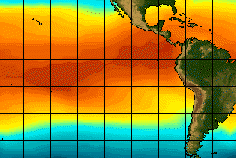Search IntMath
Close

# Systems of EquationsThe 1997-8 El Nino ...
Warmest ocean surface areas in red

The El Nino occurs every few years due to an increase in the sea temperature to the west of South America. The El Nino causes extreme weather around the globe, including droughts in Australia and Asia.

Climatologists use very interesting mathematics to try to predict this event.

Modelling changes in the climate requires an understanding of many types of equations, including simultaneous equations.

In this chapter, we see how to solve simple sets of linear equations and go on to solve pairs of non-linear equations.

## In this Chapter

We begin with the definition of simultaneous linear equations »

## Problem SolverThis tool combines the power of mathematical computation engine that excels at solving mathematical formulas with the power of GPT large language models to parse and generate natural language. This creates math problem solver thats more accurate than ChatGPT, more flexible than a calculator, and faster answers than a human tutor. Learn More.